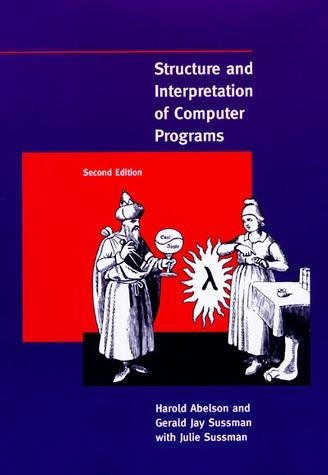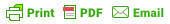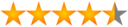# SICP summary and notesAuthors: Harold Abelson and Gerald Jay Sussman with Julie Sussman

.The sicp covers the root of programming languages and the ways to handle complexity of programs. The book is more inclined towards mathematical side of programming.

## Preface

It doesn’t matter much what the programs are about or what applications they serve. What does matter is how well they perform and how smoothly they fit with other programs in the creation of still greater programs. The programmer must seek both perfection of part and adequacy of collection. In this book the use of “program” is focused on the creation, execution, and study of programs written in a collection.

Focus on 3 phenomena

1. Human mind
1. Collection of programs
1. The computer

### Evolution of computer program

A program evolves according to the model which grows based on our understanding. A program reaches a metastable state where it stabilizes.

### Correctness of the program

• Adequacy, correctness and consistency must be verified for the small program as it is cumbersome to do the same for large scale software.
• Such small programs are called idioms which can be organized into larger programs using several organizational techniques.

### Algorithms

• performs a precise mathematical functions.
• Optimality depends on execution time and storage requirements(space)

### Role of language

• A language does not only instructs a computer to perform the task but also a way to express ideas regarding methodology.

Program must be written for people to read and only accidently for computers to execute.

Computer science” is not a science and that its significance has little to do with computers. The computer revolution is a revolution in the way we think and in the way we express what we think. The essence of this change is the emergence of what might best be called procedural epistemology —the study of the structure of knowledge from an imperative point of view, as opposed to the more declarative point of view taken by classical mathematical subjects.

Mathematics deals with “what is.”(declarative) and computation with “how to.”(Imperative)

## 1. Building Abstractions with Procedures

### Process

• Abstract
• manipulates data.
• Programs(pattern of rules) direct process.
• Programs need high accuracy.
• Programmers must learn to program for accuracy and must understand the consequences of unintended bugs or errors.
• Real world Programming requires.
1. Care
1. Expertise
1. Wisdom
• For example, programming an airplane requires all of these to avoid small bugs which can lead to catastrophic failures.

#### Qualities of a master programmer

• Ability to organize program so that resulting process will perform the task as intended.
• Can visualize the behavior of the software in advance.
• Can debug when problems arise.

well designed programs are designed in modular parts.

### The Elements of Programming

A programming language serves two functions.

• instruct computer to perform a task.
• helps to organize our ideas about the process.
• (ways to combine simple ideas to complex)

Each language has 3 parts to achieve this

1. Primitive
• Simplest entity (Eg. primitive data types(int), primitive functions (+,-))
1. Ways of combination
• Compound elements from several simple and compound elements.
1. Means of abstraction
• means by which compound element can be named and manipulated as units. (Class, Hierarchy, functions)

### Two kinds of elements

• We deal with
1. Data
• Stuff we want to manipulate
1. Procedure
• description of rules to manipulate the data.

At the basic level,the procedure are not so distinct from data.

### Expressions

• Expression is a statement.
• A primitive expression
• Eg. Numbe
• Compound expression
• (+ 4 3) two primitive expression are combined to build a compound expression.

### Combination

• Begins and ends with parenthesis.
• Has one or more expressions.
(+        4      3)
^        ^
|        |
Operator  Operand


• Prefix notation: The operator before the operand.
• allows nesting of expressions as the operator is already defined on the left side.
• non-confusing as the operator is the first element in the combination.

### Naming and the Environment

The environment plays a significant role as it allows to store the symbol and related instructions.

1. Global
1. Local

### Evaluating Combinations

• Combination evaluation is recursive in nature.

To evaluate a combination - 1. Evaluate sub-expression - 2. Apply procedure by replacing parameters with arguments in its body.

(_+ (+1 2)_ 3)

can be visualized as a tree

|
/   \
/|\    3
+ 1 2



The compound expressions simplifies to a primitive expression which can be evaluated solving.

1. the value of number(primitive expression) as the name .
2. primitive procedure symbol with equivalent machine instruction
3. other symbols replaced by their value in the environment.
The 2nd rule is a form of 3rd rule as the primitive symbol's meaning depends on its value in the global environment.

### special form and evaluation rules

• Each special form is handled using specific evaluation rules for them
• For ex. the form (define x 3) is evaluated by associating x with value 3 in the environment.

### Compound Procedures

(define <name> <formal parameter>)
(<body>)


The compound procedure are a unit of abstraction. name is associated with the body of the procedure.

### The Substitution Model for Procedure Application (Applicative Order)

It is only a descriptive model to make you understand how evaluation works. For language based on OOPS, the evaluation model becomes complex.

#### Rules

To apply a compound procedure to arguments, evaluate the body of the procedure with each formal parameter replaced by the corresponding argument.

in practice, substitution model is evaluated using local environment for the formal parameters.

### Normal order substitution model

• Fully expand and then reduce model
• Evaluates the operator until we obtain primitive procedures and then evaluate the value by replacing formal parameter with arguments.

Both can provide different result depending on how the compound procedure is structured.

Ex.

define (p (p))
(define zero (x y)
(if x = 0) x y)
)

(zero 0 p)

Here, normal order would not try to evaluate p in the beginning and would fully expand the operator whereas Applicative order would throw the error as p is not defined correctly.


### Conditional Expressions and Predicates

Similar to mathematical case analysis

|x|   = { x if x >0
{ 0 if x = 0
{ -x if x < 0


(define (abs x)
(
(cond (> x 0 ) x)
((= x 0) 0)
((< x 0) -x)))



### Local names

• The meaning of a procedure should be independent from the names used for formal parameter.
• Eg. (define (square x) (* x x)) should be similar to (define (square y) (* y y))

#### Bounded variable

• The formal parameter are bounded to the procedure definition.
• The meaning of procedure definition remains unchanged even when all occurance of formal parameter is changed.

#### Free variable

• These are unbounded variable. The meaning of the procedure changes on change of the free variable.
• Eg. If we change * in square to +, then the meaning changes.

#### Scope

• Scope defines the boundary for a name. The binding define the scope.
• In case of procedure, the scope of local variable is limited to procedure body.

### Internal definition and Block structure

• Block structure -> it is the nested definition which means definition inside a definiton.

For example,

(define ( square-square x)
(define (square  x)* x x)
(square x)
)

• Why use block structure.
• Helps to break large program in tractable pieces which eases organization of large software.

### 1.2 Procedures and the Processes they Generate

A procedure is a a pattern for local evolution of a computational process. It specifies how each stage of process is built on the previous one.

#### Linear Recursion and Iteration

We can generalize the procedural pattern in both cases.

##### Linear recursion

factorial takes O(n) space and time

(factorial 3)
(* 3 (factorial 2))
(* 3(* 2 (factorial 1)))
(* 3(* 2 1)))
(* 3 2))
(6)

• Called deffered chain of operations
• cannot be resumed even if the state is saved.
##### Iterative
• Takes O(n) time and O(1) space.
(factorial 6)
(fact-iter 1 1 6)
(fact-iter 1 2 6)
(fact-iter 2 3 6)
(fact-iter 6 4 6)
(fact-iter 24 5 6)
(fact-iter 120 6 6)
(fact-iter 720 7 6)
720


The iterative state has

• a fixed number of variables
• describe how the variable will be updated from state to state.
• an optional end test to terminate the procedure.
• can be resumed as the state is saved in the variables.

### Tree Recursion

• useful for hierarchical data.
             ________fib(5)_______
/                    \

fib(4)                     fib(3)
/     \                    /       \
fib(3)      fib(2)           fib(2)     fib(1)
/     \         /    \         /   \        |
/       \       /      \       /     \       1
fib(2)  fib(1)  fib(1) fib(0) fib(1)  fib(0)
/   \   |       |       |     |       |
/     \  1       1       0     1       0
fib(1) fib(0)
|        |
1        0

• Dynamic programming allows to optimize tree recursion by storing evaluated values in a table to avoid re-evaluation of same values.